# WKB approximation for Barrier Tunneling

The WKB approximation, short for Wentzel–Kramers–Brillouin approximation, is a powerful method in quantum mechanics for approximating the solutions to the Schrödinger equation. The WKB approximation is particularly useful in situations where the potential energy varies smoothly over space.

The WKB approximation is based on the idea of treating the Schrödinger equation as a classical equation of motion. This means that the wave function is assumed to vary slowly over space, which allows it to be expanded in a series of powers of a small parameter. The leading term in this expansion is known as the WKB approximation.

The WKB approximation is useful because it allows one to calculate the wave function and energy levels of a quantum mechanical system without having to solve the Schrödinger equation exactly. This can be especially helpful in cases where the Schrödinger equation is difficult or impossible to solve exactly.

The WKB approximation is valid when the de Broglie wavelength of the particle is much smaller than the characteristic length scale of the potential. In other words, it is valid when the particle behaves classically, except for small quantum corrections.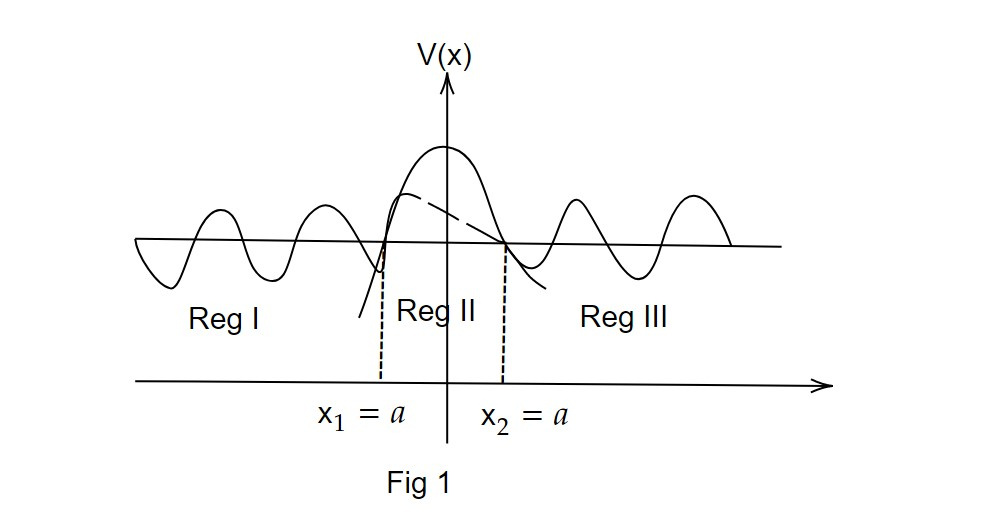Outside of the barrier, the wavefunction oscillates, whereas inside the barrier, it exhibits exponential behavior. Assume the barrier potential has the shape shown in fig 1. The two turning points x1 and x2 are located at E = v(x), where E is the total energy of the particle that was impacted against the barrier from the left.

We know that there is no probability of getting through the barrier V(x) classically. However from quantum mechanics, we find the incoming wave of amplitude A will give rise to a reflected wave of amplitude B and the transmitted wave of amplitude C.

The WKB wave function for all the regions are expressed as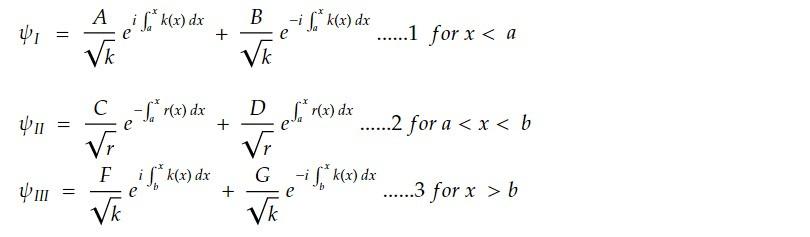As there is no reflected wave from the right G=0. At turning point x1 =a, rewriting 1 in its trigonometric form.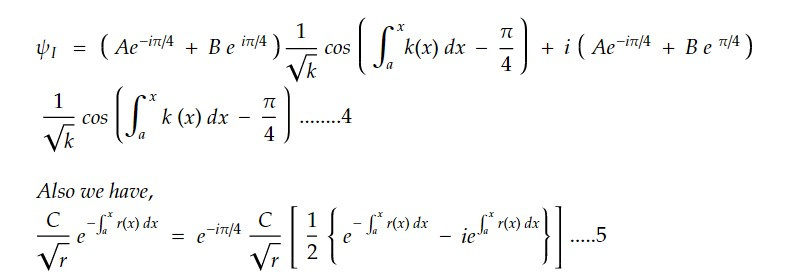On applying the boundary condition for connection formulas at x1 =a (turning point) we get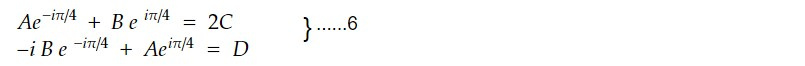Similarly, we will do the same at the next turning points x2 =b. Now, re writing by changing its limit of integrals.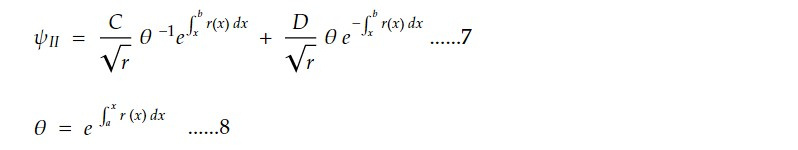Similarly using boundary condition at x2 =b, we get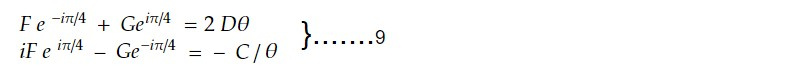Again expressing eq 6 and 9 in a simple matrix form we have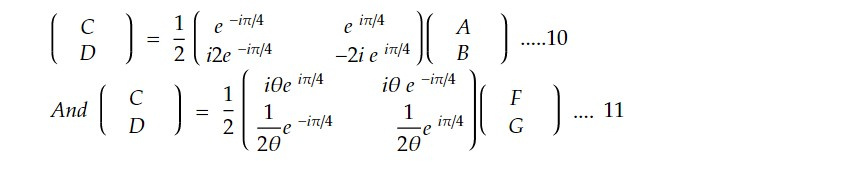Comparing equation 9 and 10 with a bit matrix algebra we get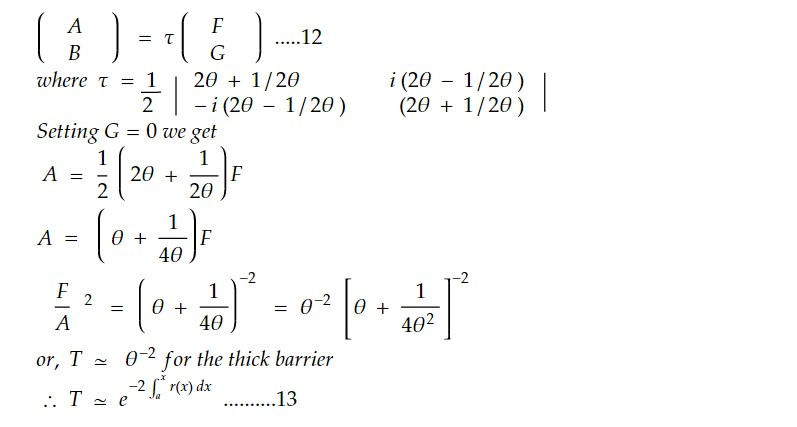Thus 𝜃 measures the opacity of the barrier.

This note is a part of the Physics Repository.